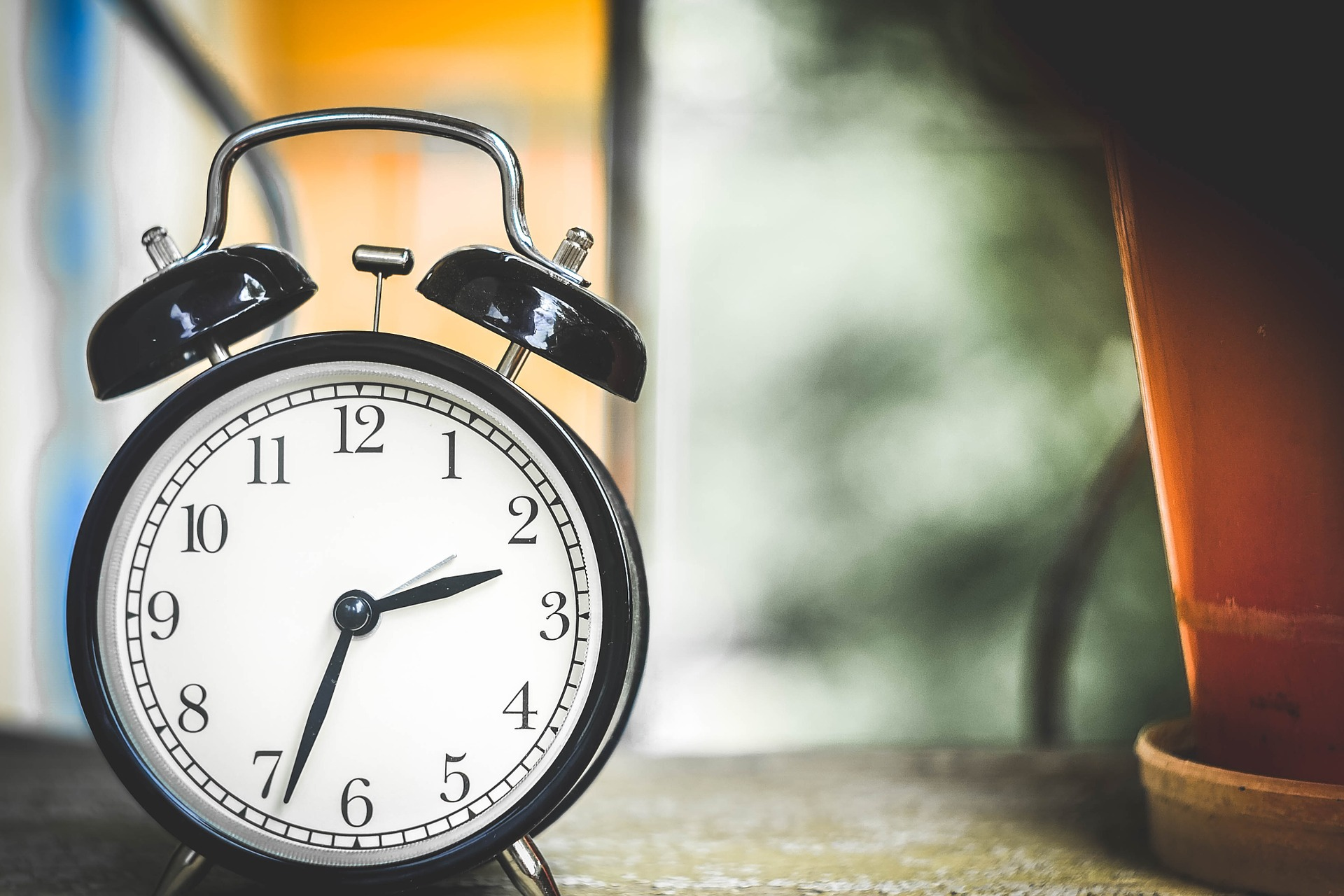# Curious Kids: Why do we count to 10?

Counting is perhaps one of the oldest scientific operations still in use today.

From a young age we learn to count the numbers 0, 1, 2, 3, 4, 5, 6, 7, 8, 9. These are called the digits. But there is a problem with ten: we have to write it differently because we used up all our digits!

What do we do now?

We use two digits. The number 10 has a left digit “1” which has a new meaning. It represents the number of times we ran out of digits. The right digit “0” is the same as before and lets us continue counting again. Mathematicians call this a place-value number system, and counting in tens is called the decimal system. Australia and the UK use the decimal system to count money, distances and lots of other things we need to measure or count.

Machines also count, but not in tens.Nature gave us ten fingers, and so it is natural for us to count in tens. But machines are built using switches, so it is natural for them to count only off (0) and on (1). This is like counting on one hand that only has one finger.

Machines count bigger numbers in the same way we do: by counting how many times they run out of digits. This system is called binary and the binary number 10 means the machine ran out of digits one time. A human would call this number two.

Today, these are the main ways of counting. But they are just two different ways of doing the same thing.The big hand of a clock has 60 different digits: 0, 1, 2, all the way up to 59. But what happens when we have used up all of those digits?

Like before, we count the number of times we run out digits, and we call each one an hour. Counting in this way is called sexagesimal.

But why do we use a different measurement for time?

We inherited the sexagesimal system from the Sumerians more than 4,000 years ago. It has lasted for so long because you can easily divide a number into two, three, four, five or six equal parts. Try dividing an hour into three equal parts and you will see there are 20 minutes each. Now try dividing a dollar into three equal parts and you will see there are 33, 33 and 34 cents each.

Our world uses many different place-value number systems, and they are all useful for different reasons.

Source The ConversationDaniel Mansfield

Daniel Mansfield is an Associate Lecturer in Mathematics, UNSW Sydney